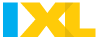AlgebraBig Ideas Algebra I
Log in through Clever:
User name - last name, first initial
Password - bird month, day and four digit year

Extra Credit - open the document below:

Currently we are learning about Solving Linear Equations

Up next.... Solving Linear Inequalities

Important Dates:
Chapter 1 Quiz Algebra A 9/25, Algebra B 9/24
Chapter 1 Test Algebra A 10/28, Algebra B 10/27All in Algebra 1 section

Check Google Classroom for IXL assignments.
45 minutes per week are required.

Thinking ahead...Triton EOC assessment topics:
Solving multi step equations and justifying steps
Solving systems of linear equations
Solve absolute value equations
Use quadratic formula
completing the square
Finds slope given two points
Write equations in slope-intercept form
Identify axis of symmetry, vertex, find domain and range, and x-intercepts
of a quadratic function and graph it
Graph a linear function
Solve quadratic equations (factoring, grouping, etc....)
Identify the number of solutions of an equations
Solve an equation for a given variable
Find x and y-intercept of a linear equation given to you in standard form
Pythagorean Theorem
Function Notation
Parallel and perpendicular lines
Inequalities
compound inequalities (and/or)
Vertex form of absolute value equation
simplify expressions using power rules
identify a function from a table (quadratic, linear, exponential)
Exponential decay
graph and identify systems of linear inequalities (shading, test point)
subtract polynomials (distribute the negative)
Factor polynomials (pull out GCF)
Use Foil to simplify
Real life situation involving a quadratic (given an equation, substitute in)
Find solutions, zeros, x-intercepts, roots of a quadratic
Find axis of symmetry of a quadratic
Find solutions of a quadratic from a graph
Find the value of the discriminant
Identify a linear equation from a group of equations
Simplify a radical expression
Match a radical equation to a graph (translations)
Identify vertex of quadratic in vertex form
Factor a polynomial by grouping